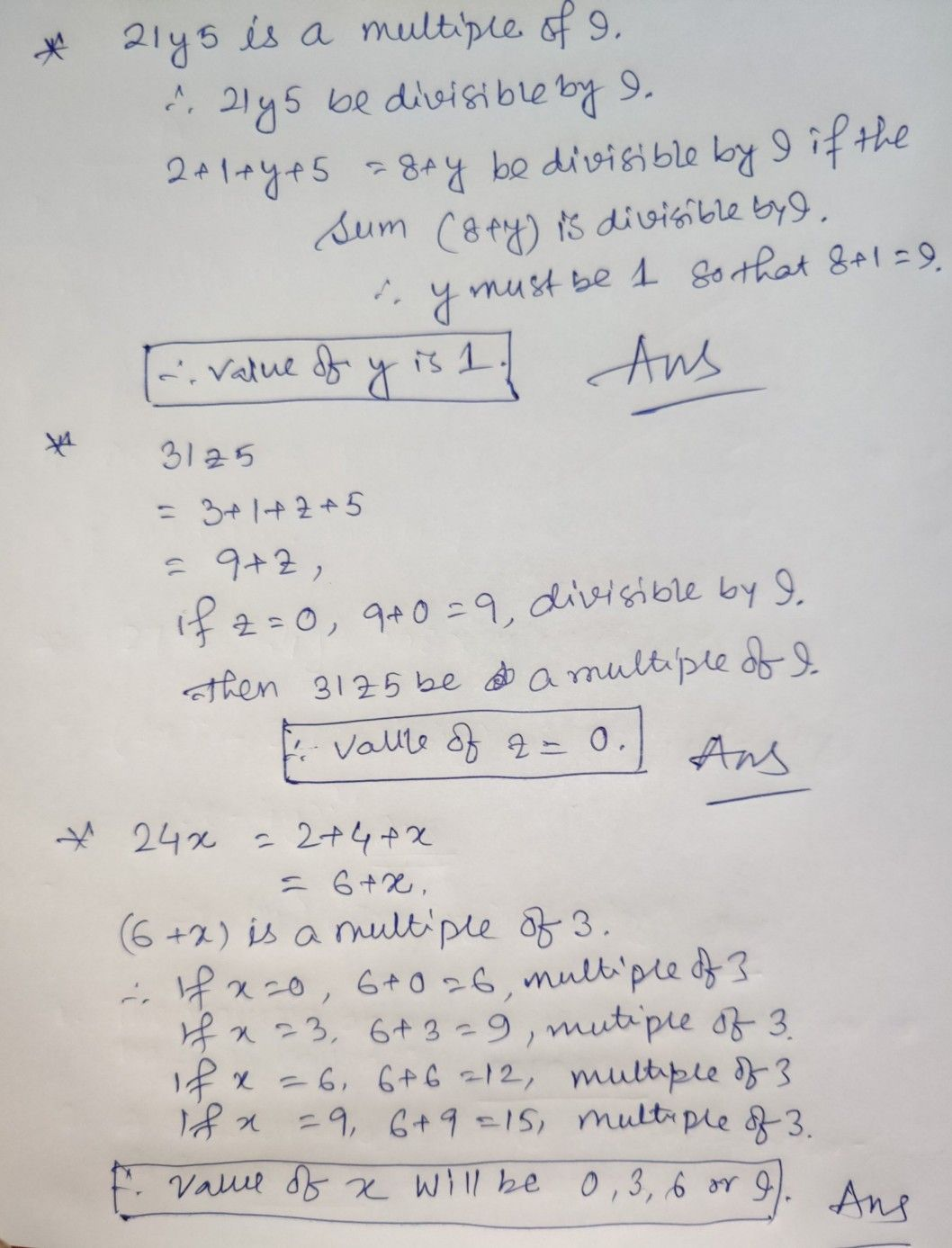Symbol
Problem$○hcckbhc$ $a1y1s10$ 1. 108 2. $616$ EXERCISE $I.2$ 1. $ff2ly5$ is a multiple of 9, where y is a digit, what is the value of y? 2. $If31z5is$ a multiple of9, where z is a digit, what is the value of w z? You will find that there are two answers fIor the last problem $NAy$ 3. $If24xisam$ multiple of 3, where x is a digit, what is the value of x? (Since $24xisam$ multiple $of3,$ its sum of digits $6+xisam$ multiple of of these numbers: $0,3,6,9,$ $12,15,18$ ... . But since x is a digit, i $6+x=6or9$ or 12 or $15.$ Therefore, $x=0or3$ or 6 or 9. Thus, = four different values.) $c3pz5$ is a $multip|e$ of 3, where z is a digit, what might be the ve
7th-9th grade
Algebra
SolutionQanda teacher - IshitaIf you have any follow up question, kindly aske me Dear Student.